# Atkins de Paula Atkins Physical Chemistry 9 e

• Slides: 23Atkins & de Paula: Atkins’ Physical Chemistry 9 e Chapter 20: Molecules in Motion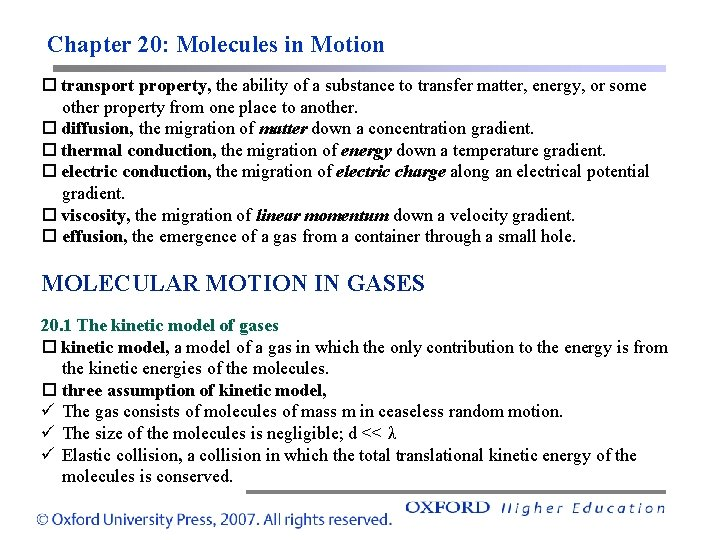Chapter 20: Molecules in Motion transport property, the ability of a substance to transfer matter, energy, or some other property from one place to another. diffusion, the migration of matter down a concentration gradient. thermal conduction, the migration of energy down a temperature gradient. electric conduction, the migration of electric charge along an electrical potential gradient. viscosity, the migration of linear momentum down a velocity gradient. effusion, the emergence of a gas from a container through a small hole. MOLECULAR MOTION IN GASES 20. 1 The kinetic model of gases kinetic model, a model of a gas in which the only contribution to the energy is from the kinetic energies of the molecules. three assumption of kinetic model, ü The gas consists of molecules of mass m in ceaseless random motion. ü The size of the molecules is negligible; d << λ ü Elastic collision, a collision in which the total translational kinetic energy of the molecules is conserved.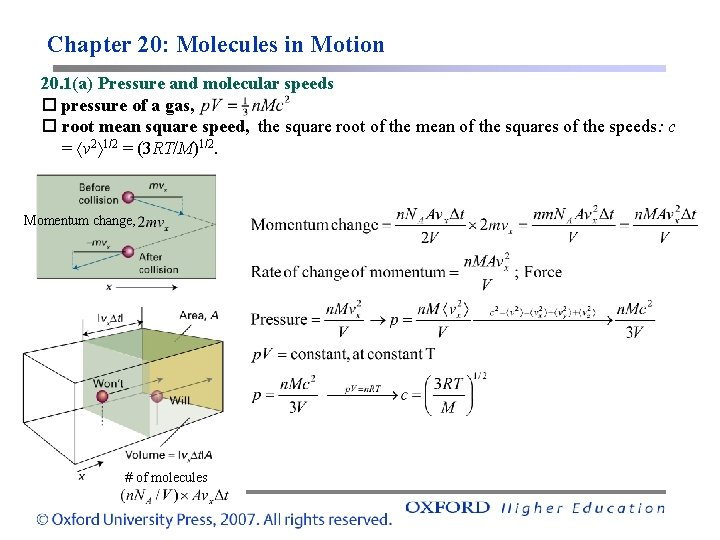Chapter 20: Molecules in Motion 20. 1(a) Pressure and molecular speeds pressure of a gas, root mean square speed, the square root of the mean of the squares of the speeds: c = v 2 1/2 = (3 RT/M)1/2. Momentum change, # of molecules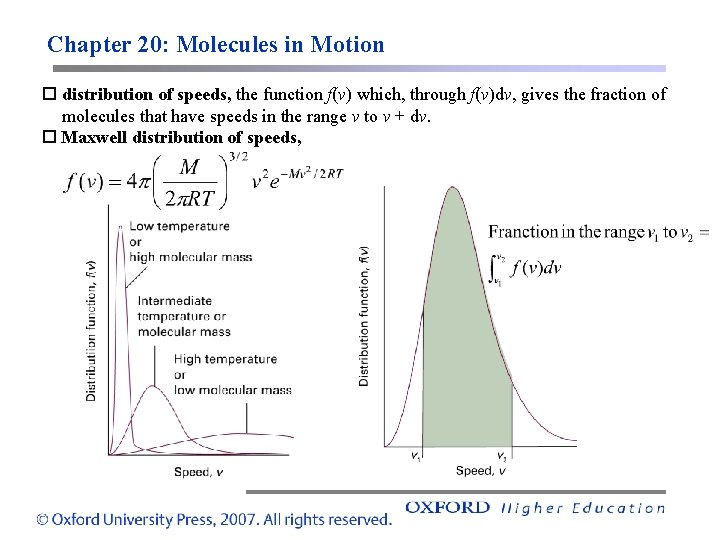Chapter 20: Molecules in Motion distribution of speeds, the function f(v) which, through f(v)dv, gives the fraction of molecules that have speeds in the range v to v + dv. Maxwell distribution of speeds,Chapter 20: Molecules in Motion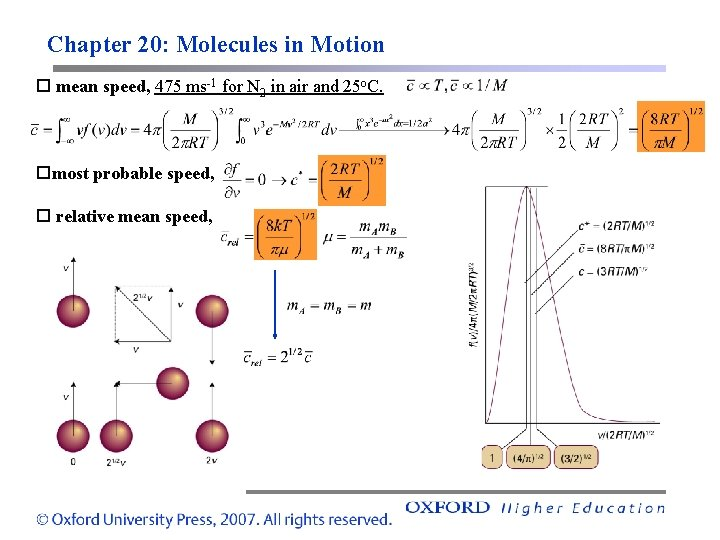Chapter 20: Molecules in Motion mean speed, 475 ms-1 for N 2 in air and 25 o. C. most probable speed, relative mean speed,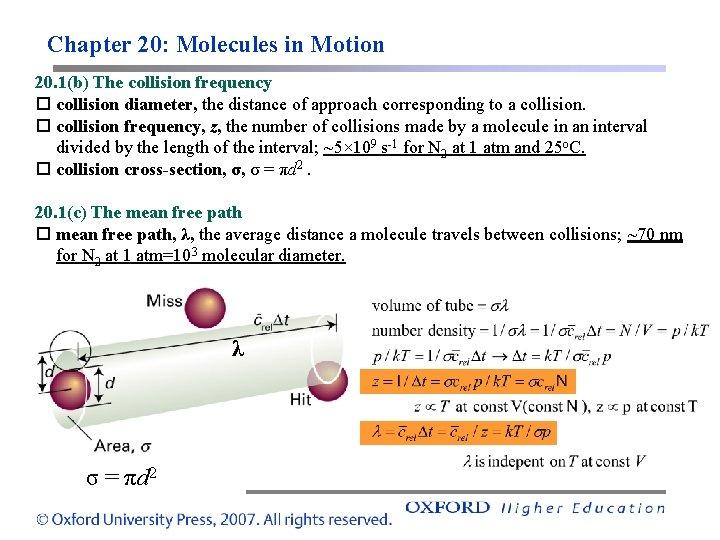Chapter 20: Molecules in Motion 20. 1(b) The collision frequency collision diameter, the distance of approach corresponding to a collision frequency, z, the number of collisions made by a molecule in an interval divided by the length of the interval; ~5× 109 s-1 for N 2 at 1 atm and 25 o. C. collision cross-section, σ, σ = πd 2. 20. 1(c) The mean free path, λ, the average distance a molecule travels between collisions; ~70 nm for N 2 at 1 atm=103 molecular diameter. λ σ = πd 2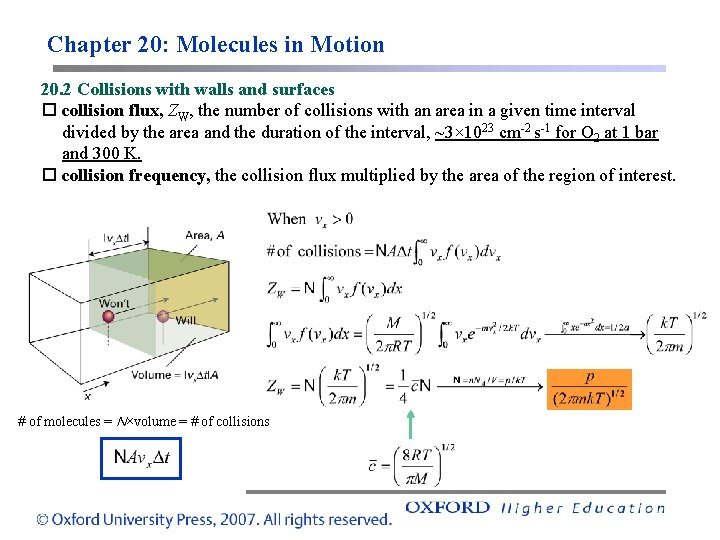Chapter 20: Molecules in Motion 20. 2 Collisions with walls and surfaces collision flux, ZW, the number of collisions with an area in a given time interval divided by the area and the duration of the interval, ~3× 1023 cm-2 s-1 for O 2 at 1 bar and 300 K. collision frequency, the collision flux multiplied by the area of the region of interest. # of molecules = N×volume = # of collisions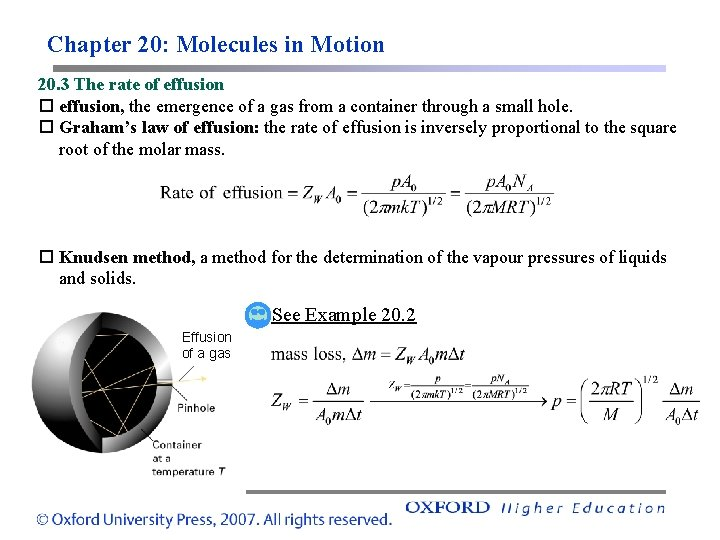Chapter 20: Molecules in Motion 20. 3 The rate of effusion, the emergence of a gas from a container through a small hole. Graham’s law of effusion: the rate of effusion is inversely proportional to the square root of the molar mass. Knudsen method, a method for the determination of the vapour pressures of liquids and solids. See Example 20. 2 Effusion of a gasChapter 20: Molecules in Motion 20. 4 Transport properties of a perfect gas flux, the quantity of a property passing through a given area in a given time interval divided by the area and the duration of the interval. matter flux, the flux of matter, J(matter) d. N/dz [m-2 s-1]. energy flux, the flux of energy, J(energy) d. T/dz [Jm-2 s-1]. Fick’s first law of diffusion: the flux of matter is proportional to the concentration gradient, J(matter) = –Dd. N/dz; D: diffusion coefficient. See Further information 20. 1 coefficient of thermal conductivity, κ, the coefficient κ in J(energy) = –κd. T/dz.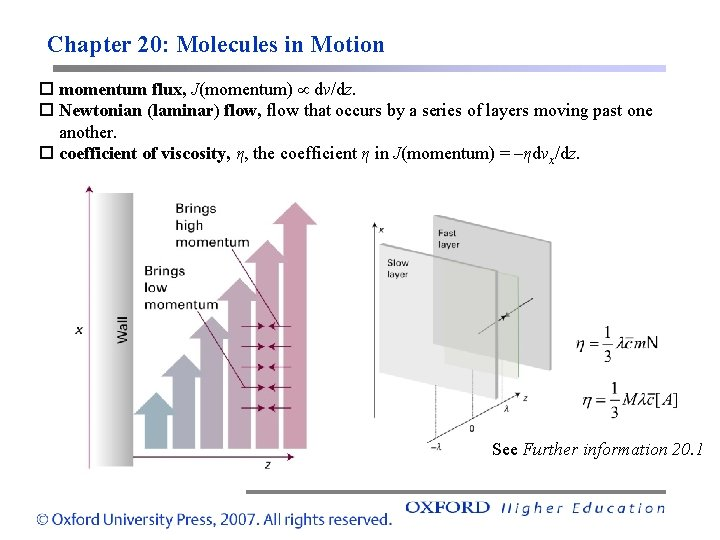Chapter 20: Molecules in Motion momentum flux, J(momentum) dv/dz. Newtonian (laminar) flow, flow that occurs by a series of layers moving past one another. coefficient of viscosity, η, the coefficient η in J(momentum) = –ηdvx/dz. See Further information 20. 1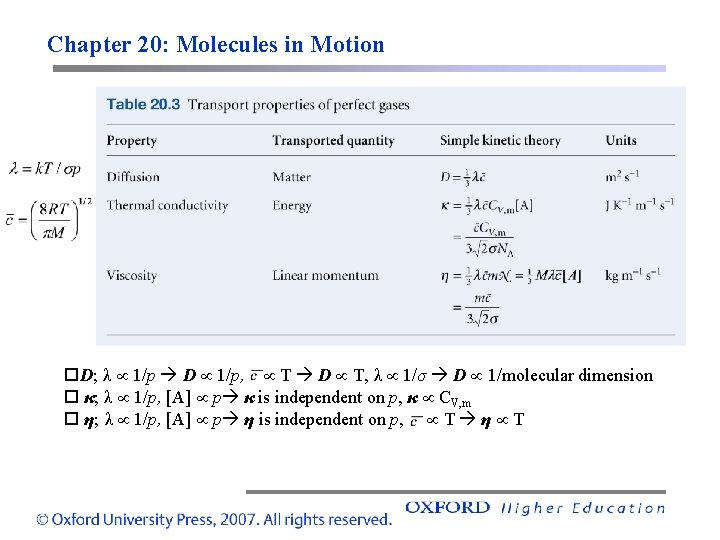Chapter 20: Molecules in Motion D; λ 1/p D 1/p, T D T, λ 1/σ D 1/molecular dimension κ; λ 1/p, [A] p κ is independent on p, κ CV, m η; λ 1/p, [A] p η is independent on p, T η TChapter 20: Molecules in Motion MOLECULAR MOTION IN LIQUIDS 20. 5 Experimental results NMR, EPR, inelastic neutron scattering, viscosity measurements, study on the molecular motion in liquids. viscosity measurements, η e. Ea/RT (mobility of the particles e-Ea/RT )Chapter 20: Molecules in Motion 20. 6 The conductivities of electrolyte solutions conductance, G, the inverse of resistance; [G]=Ω-1 or S. conductivity, the constant κ in G = κA/l; [κ]=Sm-1. molar conductivity, Λm = κ/c. strong electrolyte, an electrolyte with a molar conductivity that varies only slightly with concentration. weak electrolyte, an electrolyte with a molar conductivity that is normal at concentrations close to zero, but falls sharply to low values as the concentration increases. Kohlrausch’s law, for the concentration dependence of the molar conductivity of a strong electrolyte, Λm = Λm – Kc 1/2. limiting molar conductivity, Λm , the molar conductivity at zero concentration. law of the independent migration of ions, Λm = v+λ+ + v–λ–; λ+ and λ– are the limiting molar conductivity of cations and anions, respectively, v+ and v– are the numbers of cations and anions per formula unit of electrolyte (v+ = v– = 1 for HCl, Cu. SO 4, v+ = 1 and v– = 2 for Mg. Cl 2).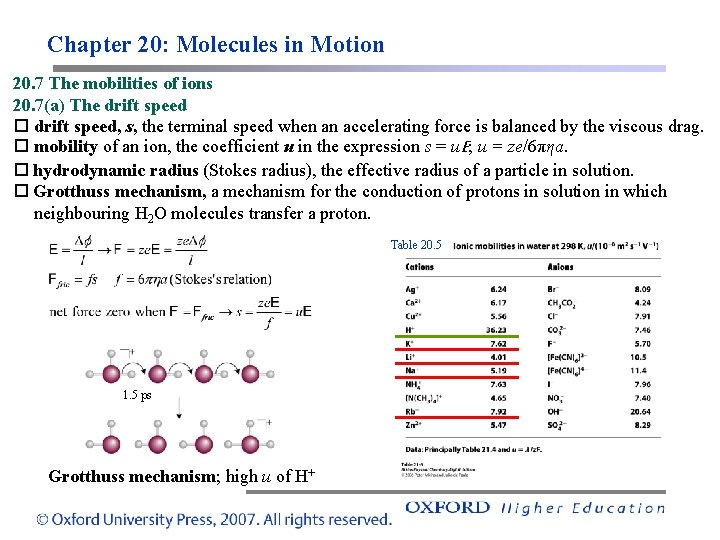Chapter 20: Molecules in Motion 20. 7 The mobilities of ions 20. 7(a) The drift speed, s, the terminal speed when an accelerating force is balanced by the viscous drag. mobility of an ion, the coefficient u in the expression s = u. E; u = ze/6πηa. hydrodynamic radius (Stokes radius), the effective radius of a particle in solution. Grotthuss mechanism, a mechanism for the conduction of protons in solution in which neighbouring H 2 O molecules transfer a proton. Table 20. 5 1. 5 ps Grotthuss mechanism; high u of H+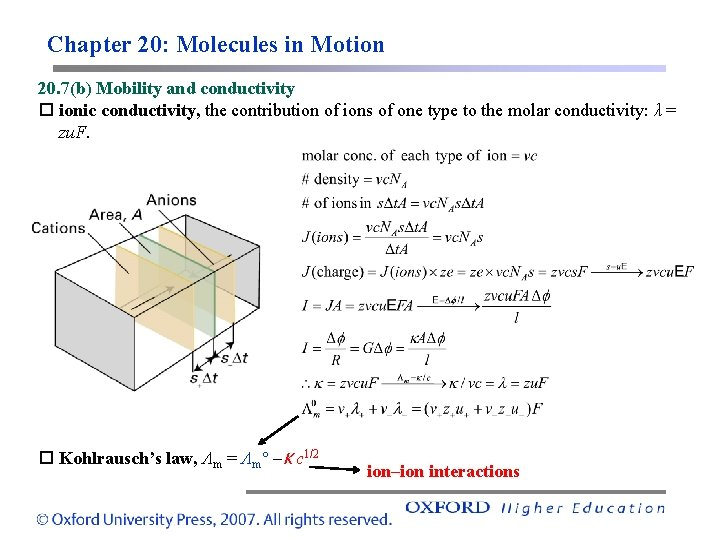Chapter 20: Molecules in Motion 20. 7(b) Mobility and conductivity ionic conductivity, the contribution of ions of one type to the molar conductivity: λ = zu. F. Kohlrausch’s law, Λm = Λm –K c 1/2 ion–ion interactions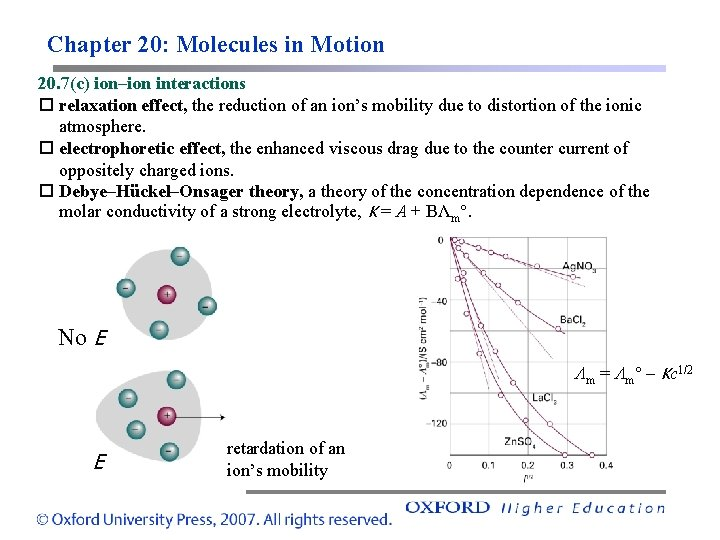Chapter 20: Molecules in Motion 20. 7(c) ion–ion interactions relaxation effect, the reduction of an ion’s mobility due to distortion of the ionic atmosphere. electrophoretic effect, the enhanced viscous drag due to the counter current of oppositely charged ions. Debye–Hückel–Onsager theory, a theory of the concentration dependence of the molar conductivity of a strong electrolyte, K = A + BΛm. No E Λm = Λm – Kc 1/2 E retardation of an ion’s mobility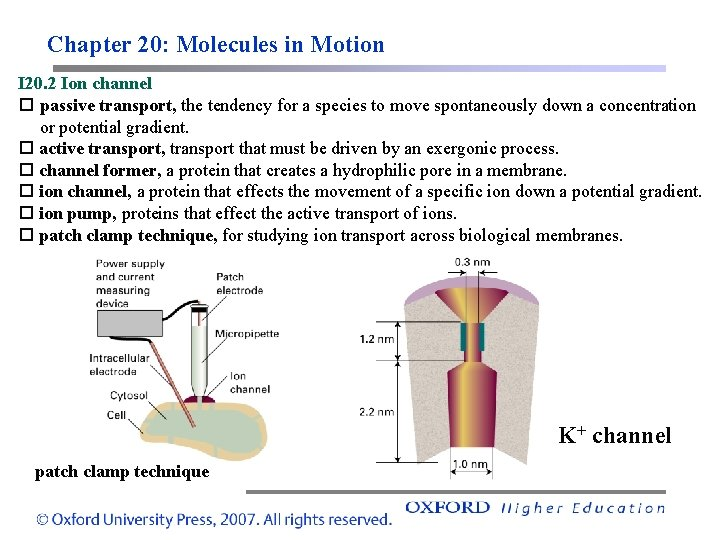Chapter 20: Molecules in Motion I 20. 2 Ion channel passive transport, the tendency for a species to move spontaneously down a concentration or potential gradient. active transport, transport that must be driven by an exergonic process. channel former, a protein that creates a hydrophilic pore in a membrane. ion channel, a protein that effects the movement of a specific ion down a potential gradient. ion pump, proteins that effect the active transport of ions. patch clamp technique, for studying ion transport across biological membranes. K+ channel patch clamp technique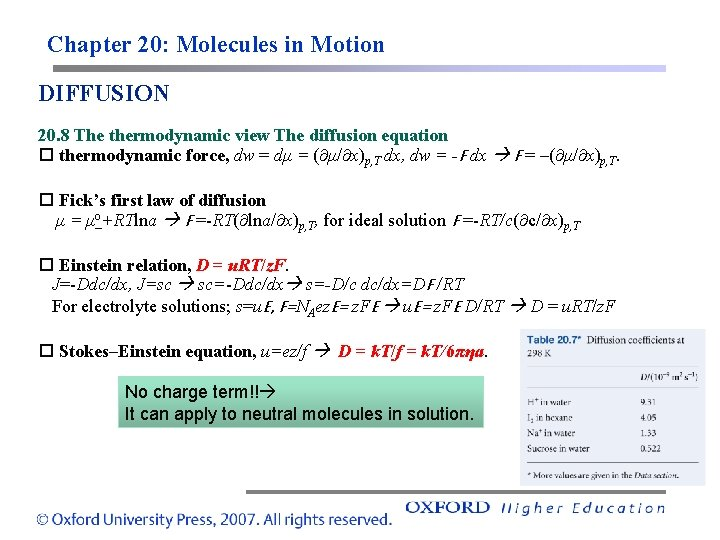Chapter 20: Molecules in Motion DIFFUSION 20. 8 The thermodynamic view The diffusion equation thermodynamic force, dw = dμ = ( μ/ x)p, T dx, dw = -F dx F = –( μ/ x)p, T. Fick’s first law of diffusion μ = μo+RTlna F =-RT( lna/ x)p, T, for ideal solution F =-RT/c( c/ x)p, T Einstein relation, D = u. RT/z. F. J=-Ddc/dx, J=sc sc=-Ddc/dx s=-D/c dc/dx=DF /RT For electrolyte solutions; s=u. E, F=NAez. E= z. FE u. E= z. FE D/RT D = u. RT/z. F Stokes–Einstein equation, u=ez/f D = k. T/f = k. T/6πηa. No charge term!! It can apply to neutral molecules in solution.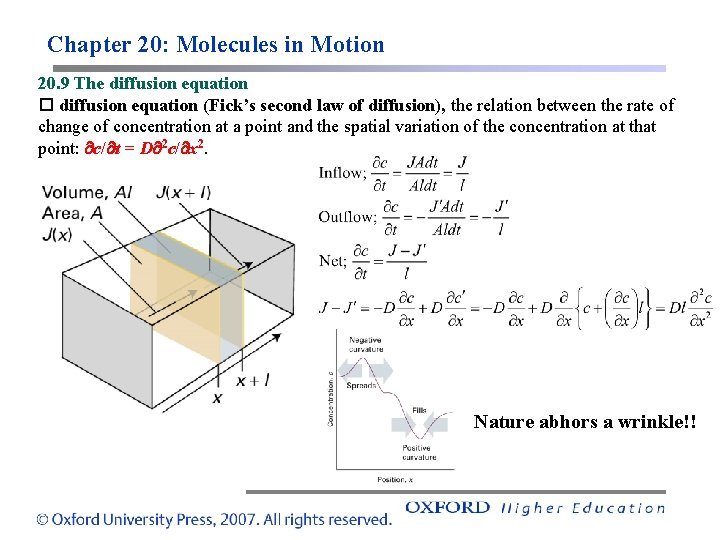Chapter 20: Molecules in Motion 20. 9 The diffusion equation (Fick’s second law of diffusion), the relation between the rate of change of concentration at a point and the spatial variation of the concentration at that point: c/ t = D 2 c/ x 2. Nature abhors a wrinkle!!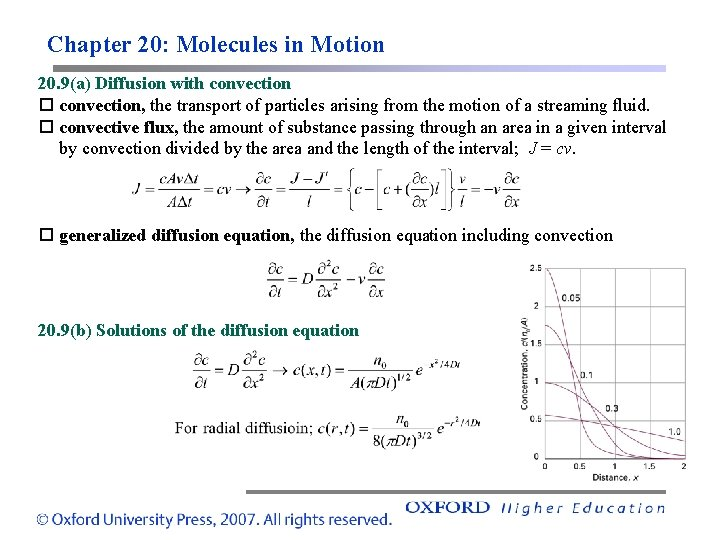Chapter 20: Molecules in Motion 20. 9(a) Diffusion with convection, the transport of particles arising from the motion of a streaming fluid. convective flux, the amount of substance passing through an area in a given interval by convection divided by the area and the length of the interval; J = cv. generalized diffusion equation, the diffusion equation including convection 20. 9(b) Solutions of the diffusion equation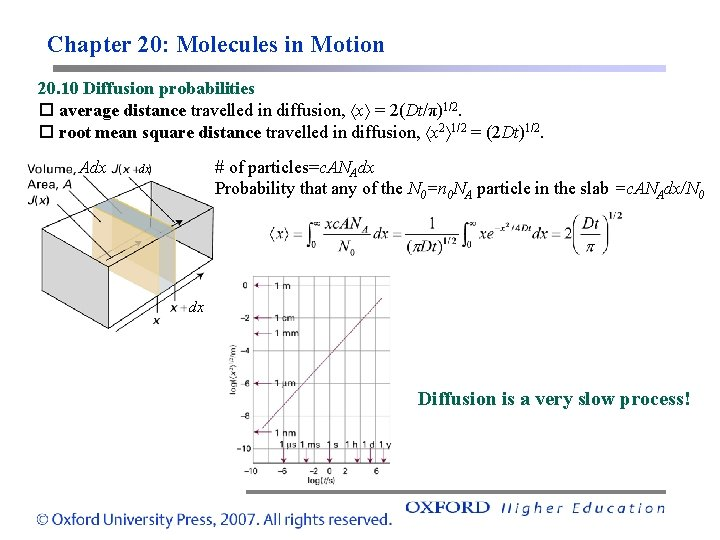Chapter 20: Molecules in Motion 20. 10 Diffusion probabilities average distance travelled in diffusion, x = 2(Dt/π)1/2. root mean square distance travelled in diffusion, x 2 1/2 = (2 Dt)1/2. Adx # of particles=c. ANAdx Probability that any of the N 0=n 0 NA particle in the slab =c. ANAdx/N 0 dx dx Diffusion is a very slow process!Chapter 20: Molecules in Motion Impact on Nanotechnology; DLA diffusion limited aggregation (DLA) Formation of nanoporous membranes through DLA process S. W. Han et al. , J. Mater. Chem. 2008, 18, 2208.